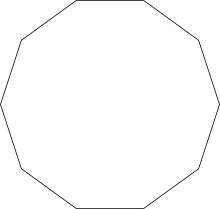# Program to Calculate the Perimeter of a Decagon

Given sideof a Decagon, the task is to find out the Perimeter of the Decagon.

Explanation : In geometry, a decagon is a ten-sided polygon or 10-gon. A regular decagon has all sides of equal length and each internal angle will always be equal to 144 degrees.Examples:

```Input : S = 5
Output : The Perimeter of Decagon is : 50

Input : S = 8
Output : The Perimeter of Decagon is : 80
```

## Recommended: Please try your approach on {IDE} first, before moving on to the solution.

The formula for finding the Perimeter of the Decagon is:

```Perimeter = 10 * S

where "S" is the side of the Decagon
```

Below is the program to find perimeter of decagon:

## C++

 `// C++ program to Calculate the Perimeter of a Decagon ` `#include ` `using` `namespace` `std; ` ` `  `// Function for finding the perimeter ` `void` `CalPeri() ` `{ ` `    ``int` `s = 5, Perimeter; ` ` `  `    ``Perimeter = 10 * s; ` ` `  `    ``cout << ``"The Perimeter of Decagon is : "` `                                ``<< Perimeter; ` `} ` ` `  `// Driver code ` `int` `main() ` `{ ` `    ``CalPeri(); ` ` `  `    ``return` `0; ` `} `

## C

 `// C program to Calculate the Perimeter of a Decagon ` `#include ` ` `  `// Function for finding the perimeter ` `void` `CalPeri() ` `{ ` `    ``int` `s = 5, Perimeter; ` ` `  `    ``Perimeter = 10 * s; ` ` `  `    ``printf``(``"The Perimeter of Decagon is : %d"``,  ` `                                    ``Perimeter); ` `} ` ` `  `// Driver code ` `int` `main() ` `{ ` `    ``CalPeri(); ` ` `  `    ``return` `0; ` `} `

## Java

 `/*package whatever //do not write package name here */` `// Java program to Calculate the Perimeter of a Decagon ` ` `  `import` `java.io.*; ` ` `  `class` `GFG { ` ` `  `// Function for finding the perimeter  ` `static` `void` `CalPeri()  ` `{  ` `    ``int` `S = ``5``, Perimeter;  ` ` `  `    ``Perimeter = ``10` `* S;  ` ` `  `    ``System.out.println(``"The Perimeter of Decagon is : "``+ ` `                                ``Perimeter);  ` `}  ` ` `  `// Driver code  ` `    ``public` `static` `void` `main (String[] args) { ` `            ``CalPeri(); ` `    ``} ` `} ` `//This Code is contributed by ajit `

## Python3

 `# Python3 program to Calculate  ` `# the Perimeter of a Decagon ` ` `  `# Function for finding the  ` `# perimeter ` `def` `CalPeri(): ` `    ``s ``=` `5` `    ``Perimeter ``=` `10` `*` `s ` ` `  `    ``print` `(``"The Perimeter of Decagon is : "` `           ``, Perimeter)  ` ` `  `# Driver code ` `if` `__name__ ``=``=` `'__main__'``: ` `    ``CalPeri(); ` ` `  `# This code is contributed by ` `# Surendra_Gangwar `

## C#

 `// C# program to Calculate the  ` `// Perimeter of a Decagon ` `using` `System; ` ` `  `class` `GFG ` `{ ` ` `  `// Function for finding the perimeter  ` `static` `void` `CalPeri()  ` `{  ` `    ``int` `S = 5, Perimeter;  ` ` `  `    ``Perimeter = 10 * S;  ` ` `  `    ``Console.WriteLine(``"The Perimeter of "` `+  ` `                           ``"Decagon is : "``+ ` `                                ``Perimeter);  ` `}  ` ` `  `// Driver code  ` `public` `static` `void` `Main ()  ` `{ ` `    ``CalPeri(); ` `} ` `} ` ` `  `// This Code is contributed  ` `// by anuj_67 `

## PHP

 ` `

Output:

```The Perimeter of Decagon is : 50
```

My Personal Notes arrow_drop_upCheck out this Author's contributed articles.

If you like GeeksforGeeks and would like to contribute, you can also write an article using contribute.geeksforgeeks.org or mail your article to contribute@geeksforgeeks.org. See your article appearing on the GeeksforGeeks main page and help other Geeks.

Please Improve this article if you find anything incorrect by clicking on the "Improve Article" button below.

Improved By : jit_t, vt_m, SURENDRA_GANGWAR